# Resources tagged with: Working systematically

Filter by: Content type:
Age range:
Challenge level:

### There are 130 results

Broad Topics > Thinking Mathematically > Working systematically### LCM Sudoku

##### Age 14 to 16 Challenge Level:

Here is a Sudoku with a difference! Use information about lowest common multiples to help you solve it.### Ben's Game

##### Age 11 to 16 Challenge Level:

Ben, Jack and Emma passed counters to each other and ended with the same number of counters. How many did they start with?### American Billions

##### Age 11 to 14 Challenge Level:

Play the divisibility game to create numbers in which the first two digits make a number divisible by 2, the first three digits make a number divisible by 3...### A First Product Sudoku

##### Age 11 to 14 Challenge Level:

Given the products of adjacent cells, can you complete this Sudoku?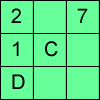### Alphabetti Sudoku

##### Age 11 to 16 Challenge Level:

This Sudoku requires you to do some working backwards before working forwards.### Cayley

##### Age 11 to 14 Challenge Level:

The letters in the following addition sum represent the digits 1 ... 9. If A=3 and D=2, what number is represented by "CAYLEY"?##### Age 11 to 14 Challenge Level:

If you take a three by three square on a 1-10 addition square and multiply the diagonally opposite numbers together, what is the difference between these products. Why?### How Old Are the Children?

##### Age 11 to 14 Challenge Level:

A student in a maths class was trying to get some information from her teacher. She was given some clues and then the teacher ended by saying, "Well, how old are they?"### Star Product Sudoku

##### Age 11 to 16 Challenge Level:

The puzzle can be solved by finding the values of the unknown digits (all indicated by asterisks) in the squares of the $9\times9$ grid.### Football Sum

##### Age 11 to 14 Challenge Level:

Find the values of the nine letters in the sum: FOOT + BALL = GAME### Product Sudoku

##### Age 11 to 16 Challenge Level:

The clues for this Sudoku are the product of the numbers in adjacent squares.### Two and Two

##### Age 11 to 16 Challenge Level:

How many solutions can you find to this sum? Each of the different letters stands for a different number.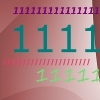### Ones Only

##### Age 11 to 14 Challenge Level:

Find the smallest whole number which, when mutiplied by 7, gives a product consisting entirely of ones.##### Age 11 to 14 Challenge Level:

Replace the letters with numbers to make the addition work out correctly. R E A D + T H I S = P A G E##### Age 11 to 16 Challenge Level:

The items in the shopping basket add and multiply to give the same amount. What could their prices be?### Diagonal Product Sudoku

##### Age 11 to 16 Challenge Level:

Given the products of diagonally opposite cells - can you complete this Sudoku?##### Age 7 to 14 Challenge Level:

I added together some of my neighbours' house numbers. Can you explain the patterns I noticed?### Special Numbers

##### Age 11 to 14 Challenge Level:

My two digit number is special because adding the sum of its digits to the product of its digits gives me my original number. What could my number be?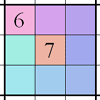### Integrated Product Sudoku

##### Age 11 to 16 Challenge Level:

This Sudoku puzzle can be solved with the help of small clue-numbers on the border lines between pairs of neighbouring squares of the grid.### Multiples Sudoku

##### Age 11 to 14 Challenge Level:

Each clue in this Sudoku is the product of the two numbers in adjacent cells.### Factor Lines

##### Age 7 to 14 Challenge Level:

Arrange the four number cards on the grid, according to the rules, to make a diagonal, vertical or horizontal line.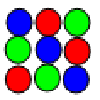### Latin Squares

##### Age 11 to 18

A Latin square of order n is an array of n symbols in which each symbol occurs exactly once in each row and exactly once in each column.### Cinema Problem

##### Age 11 to 14 Challenge Level:

A cinema has 100 seats. Show how it is possible to sell exactly 100 tickets and take exactly £100 if the prices are £10 for adults, 50p for pensioners and 10p for children.### Cuboids

##### Age 11 to 14 Challenge Level:

Can you find a cuboid that has a surface area of exactly 100 square units. Is there more than one? Can you find them all?### Peaches Today, Peaches Tomorrow...

##### Age 11 to 14 Challenge Level:

A monkey with peaches, keeps a fraction of them each day, gives the rest away, and then eats one. How long can his peaches last?### Pole Star Sudoku 2

##### Age 11 to 16 Challenge Level:

This Sudoku, based on differences. Using the one clue number can you find the solution?### Reach 100

##### Age 7 to 14 Challenge Level:

Choose four different digits from 1-9 and put one in each box so that the resulting four two-digit numbers add to a total of 100.### LCM Sudoku II

##### Age 11 to 18 Challenge Level:

You are given the Lowest Common Multiples of sets of digits. Find the digits and then solve the Sudoku.### Making Maths: Double-sided Magic Square

##### Age 7 to 14 Challenge Level:

Make your own double-sided magic square. But can you complete both sides once you've made the pieces?### Charitable Pennies

##### Age 7 to 14 Challenge Level:

Investigate the different ways that fifteen schools could have given money in a charity fundraiser.### Summing Consecutive Numbers

##### Age 11 to 14 Challenge Level:

15 = 7 + 8 and 10 = 1 + 2 + 3 + 4. Can you say which numbers can be expressed as the sum of two or more consecutive integers?### Number Daisy

##### Age 11 to 14 Challenge Level:

Can you find six numbers to go in the Daisy from which you can make all the numbers from 1 to a number bigger than 25?### Coins

##### Age 11 to 14 Challenge Level:

A man has 5 coins in his pocket. Given the clues, can you work out what the coins are?### Crossing the Bridge

##### Age 11 to 14 Challenge Level:

Four friends must cross a bridge. How can they all cross it in just 17 minutes?### Weights

##### Age 11 to 14 Challenge Level:

Different combinations of the weights available allow you to make different totals. Which totals can you make?### Olympic Magic

##### Age 14 to 16 Challenge Level:

in how many ways can you place the numbers 1, 2, 3 … 9 in the nine regions of the Olympic Emblem (5 overlapping circles) so that the amount in each ring is the same?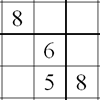### Twin Line-swapping Sudoku

##### Age 14 to 16 Challenge Level:

A pair of Sudoku puzzles that together lead to a complete solution.### Squares in Rectangles

##### Age 11 to 14 Challenge Level:

A 2 by 3 rectangle contains 8 squares and a 3 by 4 rectangle contains 20 squares. What size rectangle(s) contain(s) exactly 100 squares? Can you find them all?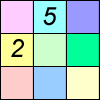### Difference Sudoku

##### Age 14 to 16 Challenge Level:

Use the differences to find the solution to this Sudoku.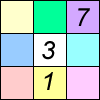##### Age 11 to 16 Challenge Level:

Four small numbers give the clue to the contents of the four surrounding cells.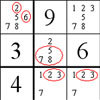### The Naked Pair in Sudoku

##### Age 7 to 16

A particular technique for solving Sudoku puzzles, known as "naked pair", is explained in this easy-to-read article.### An Introduction to Magic Squares

##### Age 7 to 16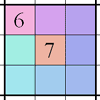### Integrated Sums Sudoku

##### Age 11 to 16 Challenge Level:

The puzzle can be solved with the help of small clue-numbers which are either placed on the border lines between selected pairs of neighbouring squares of the grid or placed after slash marks on. . . .### Spot the Card

##### Age 14 to 16 Challenge Level:

It is possible to identify a particular card out of a pack of 15 with the use of some mathematical reasoning. What is this reasoning and can it be applied to other numbers of cards?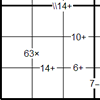### Bochap Sudoku

##### Age 11 to 16 Challenge Level:

This Sudoku combines all four arithmetic operations.### Pair Sums

##### Age 11 to 14 Challenge Level:

Five numbers added together in pairs produce: 0, 2, 4, 4, 6, 8, 9, 11, 13, 15 What are the five numbers?### Window Frames

##### Age 5 to 14 Challenge Level:

This task encourages you to investigate the number of edging pieces and panes in different sized windows.### Where Can We Visit?

##### Age 11 to 14 Challenge Level:

Charlie and Abi put a counter on 42. They wondered if they could visit all the other numbers on their 1-100 board, moving the counter using just these two operations: x2 and -5. What do you think?### Sticky Numbers

##### Age 11 to 14 Challenge Level:

Can you arrange the numbers 1 to 17 in a row so that each adjacent pair adds up to a square number?### Difference Dynamics

##### Age 14 to 18 Challenge Level:

Take three whole numbers. The differences between them give you three new numbers. Find the differences between the new numbers and keep repeating this. What happens?